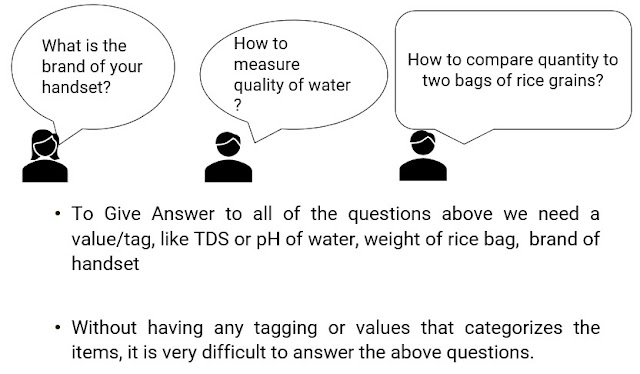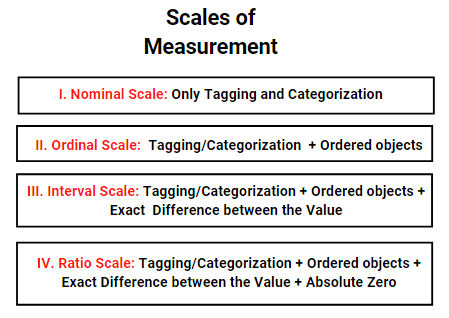# Measurement Scales

• Measurement implies measuring a property or attribute of any object, e.g. the height of Ramesh is 6 ft., here Ramesh is an object, and height is an attribute of Ramesh that is measured.
• It is the process of assigning a category or a value to an object.

## Importance of Measurement• To publish an analysis or to say how good or bad is something with respect to another thing, we need a scale to compare or categorize things on the same scale. E.g. who is the tallest boy in a class, what is the average height of the class, who is taller among Ram and Ashok etc
• Measurement Scales are rules/processes used to categorize the different types of objects e.g. Height of Ramesh, Weight of Rahul, Rank of Suresh.

## Types of Scales of Measurement

#### Nominal Scale

• It is the simplest form of a scale of measurement that simply tags an object.
• It just categorizes the data for better identification.
• It is a qualitative technique wherein no value is attached to the object by tagging it e.g. Which brand of phone do you have, the colour of the tree, etc.?

#### Ordinal Scale

• This scale categorizes the data based on order and rank.
• It assigns a comparative rank to the objects without telling the actual value associated, e.g. Ram ranks third in class, this statement tells the rank of Ram but not his marks.
• It is a qualitative technique as it doesn’t assign any values to the object.
• This scale shows the relative rankings of an object, wherein the difference between any two is not the same throughout.
• Ram ranks 1st, Raj ranks 2nd, Aman ranks 5th and Rahul ranks 6th. The difference between the ranks of Ram and Raj is the same as Aman and Ranks, but it cannot be concluded that the difference of marks between them is also the same.
• E.g. Ranking in school, Rating in Hotels/Restaurants, etc.

#### Interval Scale

• This includes all the features of the Ordinal Scale along with the exact differences between the value.
• Values between the objects can be separated to learn the actual difference between them. E.g.: Celsius Scale, Fahrenheit Scale.
• The only problem with this scale is that it cannot be used to calculate ratios due to the lack of absolute zero or in simple words they lack origin.
• Absolute Zero implies there is nothing beyond zero i.e. there is no such thing as negative, however, Celsius and Fahrenheit's scales have negative measurements as well.

#### Ratio Scale

• This is the most advanced version of scale incorporating all values of all other scales along with a clear definition of zero.
• This implies that there is a point beyond which nothing could be measured at all i.e. a point of origin.
• The best example of the Ratio Scale is the Kevin temperature scale in which there is no measurement beyond 0 Kelvin.
• There are no negative measurements on this scale as there is an absolute zero.

### Illustration of Absolute Zero and Ratio

#### Illustration 1:

• Let us take two temperatures, 20 degrees Celsius and 40 degrees Celsius.
• The ratio between the temperature is 1:2 i.e. Temperature 2 is double of temperature 1, but, the difference of heat between 20 degree Celsius and 40 Degree Celsius is way more
• Also, converting the temperatures to Fahrenheit, temperature 1 becomes 68F and temperature 2 becomes 104, here for the same temperature the ratio has changed.
• The above observation is because both scales lack Absolute Zero i.e. lack the origin temperature.

### Example of Type of Scales (Combined)

• Example 1: Ram, Rohan, Amit, and Rajesh are four students of a school, they have appeared in an all India Competitive Exam. Let us try to understand various types of Scales of Measurement form them.
Scale Ram Rohan Amit Rajesh
Nominal Roll Number 1 Roll Number 2 Roll Number 3 Roll Number 4
Ordinal Rank 3rd Rank 4th Rank 1st Rank 2nd
Interval 98 Percentile 97.5 Percentile 99..5 Percentile 99 Percentile
Ratio 95/100 marks in Maths 93/100 marks in Maths 99/100 marks in Maths 97/100 marks in Maths

### A Comparative Study of all Scales of Measurement

#### Illustration 2:

Features Nominal Scale Ordinal Scale Internal Scale Ratio Scale
Order of Value No Yes Yes Yes
Addition/Subtraction of Value No No Yes Yes
Value of Difference between two Values No No Yes Yes
Ratio No No No Yes
Absolute Zero No No No Yes
Quantitative No No Yes Yes
Qualitative Yes Yes No No

#### Illustration 3: